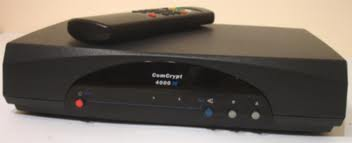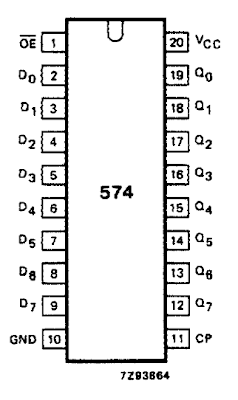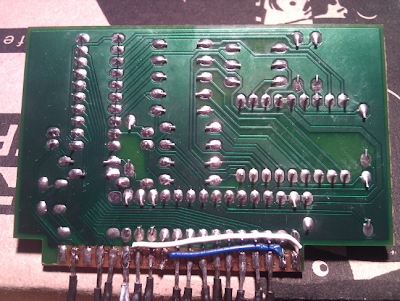## Wednesday, September 22, 2010

### The NetDuino and some 74HC574's

If you live in South Africa, you may remember these (ComCrypt 4000 MNET decoder):Well a collegue found one, ripped it apart and found this little display board inside:The board was soldered vertically onto the main board but with some judicious use of a de-soldering pump and some flux I got it free. My collegue gave it to me to have a look at.

This is how I reverse engineered it and connected it to the NetDuino:
1. First off, look at the chips on the board: we have two 74HC574 chips. A brief Google returns these as “Octal D-type flip-flop, positive edge trigger, 3-state”... at which point I went “WTF?” and had a good read on Wikipedia: http://en.wikipedia.org/wiki/Flip-flop_(electronics), then found the data sheet: http://www.datasheetcatalog.org/datasheet/philips/74HC_HCT574_CNV_2.pdf

2. Which didn’t help too much so I read that all again :)

3.4. The data sheet yielded the pinout above, and so I began tracing the connector pins to the chips and reading what the pins did. Here is a picture of the underside of the board

5.6. So.. All of the OE pins are tied to ground. This is good, it seems OE is “Output Enable”. Counting from left to right the pins are traced as:

• NC (not connected)
• Red LED GND
• NC
• GND
• Clock pin (right hand flip-flop)
• 5V (Vin)
• Clock pin (left hand flip-flop)
• Green LED GND
• GND
• INPUT 7
• INPUT 6
• INPUT 5
• INPUT 4
• INPUT 3
• INPUT 2
• INPUT 1
• INPUT 0
• GND
• 5V

7. The input lines are connected to both flip-flops on pins D0-D7. The way the flip-flop works is that you set these lines high or low and then pulse the clock to transfer the state of the inputs to the flip-flop outputs i.e. the pins connected to the 7-segment LEDs. Basically between clock pulses the flip-flops will hold their output values thus keeping the relevant bits of the 7-segment display lit.

8. From here it was a case of wiring this up to the NetDuino. I connected D0-D7 to the corresponding D0-D7 pins on the NetDuino. Clock pins went to D8 and D9, the LEDs (red and green) went to D10 and D11 and 5V and GND went to the respective 5V and GND lines on the NetDuino. (Notice above that the two 5V pins are bridged, as are the GND pins. The GND pin for one of the chips is not tied to ground and if you omit this you will get weird values on one of the 7-segment displays. I believe this is called a “floating ground” and is the source of much evil...)

A note on “Active Low” (as I understand it):

If we look at the wiring on the Green LED, we can see that the positive pin is permanently connected to a shared 5V power line (shared with the flip-flops etc.) but the negative pin is connected to a GPIO pin on the NetDuino. So how do we switch the LED on? Well we need to write false to the respective output port:

`// Set up the pinOutputPort _greenLed = new OutputPort( Pins.GPIO_PIN_D11, true );// Set the pin low_greenLed.Write( false );`

Writing false to the pin sets the GPIO port LOW i.e. brings the pin to GROUND, thereby completing the circuit and causing electricity to flow through the LED, lighting it up.

The data lines (D0-D7) are also active low, and it seems that the registration of the clock pulse occurs on the low edge of the clock. If you imagine the following (as a badly drawn square wave) then when the clock pin goes from HIGH to LOW (falling edge) the flip-flop reads the states of the input pins and transitions that state to the output pins:Okay, so I made that up, I THINK that is how it works :) If anyone can explain this better then... great!

These decoders are dirt cheap (you can pick one up for around R50-R100 (<\$15) ) and they have tons of excellent bits to harvest so go and GET EM! (The newer decoders also have bits and pieces but you have to make sure it is not a new tiny one. They are all SMD and pretty useless for our purposes). Have a look in your garage for old electronics, there is tons of stuff to harvest lying around... :) Here is the code:
`using System;using System.Threading;using Microsoft.SPOT;using Microsoft.SPOT.Hardware;using SecretLabs.NETMF.Hardware;using SecretLabs.NETMF.Hardware.Netduino;namespace Decoder{  public class Program  {   private readonly OutputPort _clockPin1;   private readonly OutputPort _clockPin2;   private readonly OutputPort _redLed;   private readonly OutputPort _greenLed;   private readonly OutputPort[] _digitPins;   private readonly byte[] _digitBits = new byte[]                                          {                                            1 + 2 + 8 + 16 + 32 + 64,                                            1 + 64,                                            2 + 1 + 4 + 16 + 32,                                            2 + 1 + 4 + 64 + 32,                                            8 + 4 + 1 + 64,                                            2 + 8 + 4 + 64 + 32,                                            2 + 8 + 16 + 32 + 64 + 4,                                            2 + 1 + 64,                                            1 + 2 + 4 + 8 + 16 + 32 + 64,                                            1 + 2 + 8 + 4 + 64,                                            128                                          };   public Program()   {     _digitPins = new OutputPort[]                    {                      new OutputPort( Pins.GPIO_PIN_D0, true ),                      new OutputPort( Pins.GPIO_PIN_D1, true ),                      new OutputPort( Pins.GPIO_PIN_D2, true ),                      new OutputPort( Pins.GPIO_PIN_D3, true ),                      new OutputPort( Pins.GPIO_PIN_D4, true ),                      new OutputPort( Pins.GPIO_PIN_D5, true ),                      new OutputPort( Pins.GPIO_PIN_D6, true ),                      new OutputPort( Pins.GPIO_PIN_D7, true ),                    };     _greenLed = new OutputPort( Pins.GPIO_PIN_D11, true );     _redLed = new OutputPort( Pins.GPIO_PIN_D10, true );     _clockPin1 = new OutputPort(Pins.GPIO_PIN_D8, true);     _clockPin2 = new OutputPort(Pins.GPIO_PIN_D9, true);   }   private void PulseClock( OutputPort pin )   {     pin.Write( false );     pin.Write( true );     pin.Write( false );   }   private void SetDigitBits( int digit )   {     if( digit < 0 || digit > 10 )       digit = 10;     digit = _digitBits[ digit ];     for( int i = 0; i < 8; i++ )     {       bool on = ( ( digit >> i ) & 1 ) == 1 ? false : true;       _digitPins[ i ].Write( !on );     }   }   private void DisplayValue( int value )   {     int high = value / 10;     int low = value - ( high * 10 );     SetDigitBits( low );     PulseClock( _clockPin2 );     SetDigitBits( high );     PulseClock( _clockPin1 );   }   private void Setup()   {     PulseClock( _clockPin1 );     PulseClock( _clockPin2 );   }   private void Loop()   {     bool greenOn = true;     int value = 0;     while( true )     {       _redLed.Write( greenOn );       _greenLed.Write( !greenOn );       greenOn = !greenOn;       for( int i = 0; i < 10; i++ )       {         DisplayValue( value++ );         Thread.Sleep( 100 );       }       if( value > 99 ) value = 0;     }   }   public static void Main()   {     Program p = new Program();     p.Setup();     p.Loop();   }  }}`